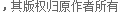import java.util.*;
import javax.servlet.http.HttpServletRequest;
private HttpServletRequest request = null; // 取得HttpServletRequest对象
private List<FileItem> items = null; // 保存全部的上传内容
private Map<String, List<String>> params = new HashMap<String, List<String>>(); // 保存所有的参数
private Map<String, FileItem> files = new HashMap<String, FileItem>();
private int maxSize = 3145728; // 默认的上传文件大小为3MB，3 * 1024 * 1024
String tempDir) throws Exception { // 传递request对象、最大上传限制、临时保存目录
this.request = request; // 接收request对象
DiskFileItemFactory factory = new DiskFileItemFactory(); // 创建磁盘工厂
if (tempDir != null) { // 判断是否需要进行临时上传目录
factory.setRepository(new File(tempDir)); // 设置临时文件保存目录
}
if (maxSize > 0) { // 如果给的上传大小限制大于0，则使用新的设置
this.maxSize = maxSize;
}
upload.setFileSizeMax(this.maxSize); // 设置最大上传大小为3MB，3 * 1024 * 1024
try {
throw e; // 向上抛出异常
}
this.init(); // 进行初始化操作
}
private void init() { // 初始化参数，区分普通参数或上传文件
Iterator<FileItem> iter = this.items.iterator();
IPTimeStamp its = new IPTimeStamp(this.request.getRemoteAddr()) ;
while (iter.hasNext()) { // 依次取出每一个上传项
FileItem item = iter.next(); // 取出每一个上传的文件
if (item.isFormField()) { // 判断是否是普通的文本参数
String name = item.getFieldName(); // 取得表单的名字
String value = item.getString(); // 取得表单的内容
List<String> temp = null; // 保存内容
if (this.params.containsKey(name)) { // 判断内容是否已经存放
temp = this.params.get(name); // 如果存在则取出
} else { // 不存在
temp = new ArrayList<String>(); // 重新开辟List数组
}
this.params.put(name, temp); // 向Map中增加内容
} else { // 判断是否是file组件
String fileName = its.getIPTimeRand()
+ "." + item.getName().split("\\.");
this.files.put(fileName, item); // 保存全部的上传文件
}
}
}
public String getParameter(String name) { // 取得一个参数
String ret = null; // 保存返回内容
List<String> temp = this.params.get(name); // 从集合中取出内容
if (temp != null) { // 判断是否可以根据key取出内容
ret = temp.get(0); // 取出里面的内容
}
return ret;
}
public String[] getParameterValues(String name) { // 取得一组上传内容
String ret[] = null; // 保存返回内容
List<String> temp = this.params.get(name); // 根据key取出内容
if (temp != null) { // 避免NullPointerException
ret = temp.toArray(new String[] {});// 将内容变为字符串数组
}
return ret; // 变为字符串数组
}
public Map<String, FileItem> getUploadFiles() {// 取得全部的上传文件
return this.files; // 得到全部的上传文件
}
public List<String> saveAll(String saveDir) throws IOException { // 保存全部文件，并返回文件名称，所有异常抛出
List<String> names = new ArrayList<String>();
if (this.files.size() > 0) {
Set<String> keys = this.files.keySet(); // 取得全部的key
Iterator<String> iter = keys.iterator(); // 实例化Iterator对象
File saveFile = null; // 定义保存的文件
InputStream input = null; // 定义文件的输入流，用于读取源文件
OutputStream out = null; // 定义文件的输出流，用于保存文件
while (iter.hasNext()) { // 循环取出每一个上传文件
FileItem item = this.files.get(iter.next()); // 依次取出每一个文件
.getIPTimeRand()
+ "." + item.getName().split("\\.");
saveFile = new File(saveDir + fileName); // 重新拼凑出新的路径
try {
input = item.getInputStream(); // 取得InputStream
out = new FileOutputStream(saveFile); // 定义输出流保存文件
int temp = 0; // 接收每一个字节
while ((temp = input.read()) != -1) { // 依次读取内容
out.write(temp); // 保存内容
}
} catch (IOException e) { // 捕获异常
throw e; // 异常向上抛出
} finally { // 进行最终的关闭操作
try {
input.close(); // 关闭输入流
out.close(); // 关闭输出流
} catch (IOException e1) {
throw e1;
}
}
}
}
return names; // 返回生成后的文件名称
}
}

• 相关链接：
• 教程说明：

JSP教程-java使用smartupload组件实现文件上传的方法